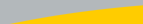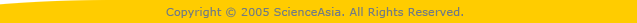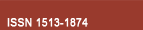| Home  | About ScienceAsia  | Publication charge  | Advertise with us  | Submit Covers  | Subscription for printed version  | Contact us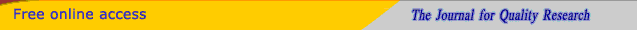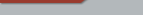Editorial Board Journal Policy Instructions for Authors Online submission Author Login Reviewer Login Web Usage Statistics Volume 48 Number 2 Volume 48 Number 1 Volume 47 Number 4 Volume 47 Number 3 Volume 47 Number 2 Earlier issuesVolume 34 Number 4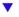Volume 35 Number 1 Volume 35 Number 2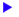Short reports

ScienceAsia 35(2009): 111-112 |doi: 10.2306/scienceasia1513-1874.2009.35.111

# Regularity-preserving elements of regular rings

## Ronnason Chinram

ABSTRACT:      Let R be a ring and aR. A variant of R with respect to a is a ring R under usual addition and multiplication o defined by x o y = xay for all x, yR. In this paper, we characterize the regularity-preserving elements of regular rings.

 Department of Mathematics, Faculty of Science, Prince of Songkla University, Hat Yai, Songkla 90112, Thailand

* Corresponding author, E-mail: ronnason.c@psu.ac.th

Received 13 May 2008, Accepted 14 Jan 2009

### INTRODUCTION

Variants of semigroups were first studied by Hickey1, although variants of concrete semigroups of relations had earlier been considered by Magil2, 3. We can see some properties of variants of semigroups in Refs. 1, 4, 5.

In this paper, we give the definition of variants of rings by using the concept of variants of semigroups and we characterize the regularity-preserving elements of regular rings.

### REGULARITY-PRESERVING ELEMENTS OF REGULAR RINGS

Let R be a ring and aR. A new product is defined on R by x y = xay for all x,yR. Then (R,+,) is a ring. We usually write (R,+,a) rather that (R,+,) to make the element a explicit. The ring (R,+,a) is called a variant of R with respect to a.

An element a of a ring R is said to be regular if there exists xR such that a = axa. A ring R is called a regular ring if every element of R is regular.

Let R be a ring. An element aR is said to be a regularity-preserving element of R if the ring (R,+,a) is regular. Denote the set of all regularity-preserving elements of R by RP(R).

Theorem 1 If R is not regular, then RP(R) is an empty set.

Proof : Assume that RP(R) is a nonempty set. Then there exists aR such that (R,+,a) is regular. Thus, for each xR, there exists yxR such that x = x yx x. Therefore, for all xR, x = xyx x = xayxax = x(ayxa)x. So x is regular in R. This implies that R is regular, a contradiction.

Question   Let R be a regular ring. Is RP(R) a nonempty set?

The author has not been able to answer this question yet. However, the following theorem is true.

Theorem 2 If RP(R) is a nonempty set, then RP(R) is a subsemigroup of (R,).

Proof : Let a,bRP(R) and xR. Then there exist y,z,s,tR such that x = xayax, x = xbzbx, a = absba, and b = batab. Thus

 x = xayax = x(absba)ya(xbzbx) = x(ab)(sbayaxbz)bx = x(ab)(sbayaxbz)(batab)x = x(ab)(sbayaxbzbat)(ab)x.
Therefore x is regular in (R,+,ab). Then abRP(R). Hence RP(R) is a subsemigroup of (R,).

Now the author studies regularity-preserving elements of regular rings having an identity.

Let R be a ring with identity 1. An element aR is called a unit of R if there exist x,yR such that ax = 1 = ya (see Ref. 6). It is easy to prove that x = y. The following theorem holds.

Theorem 3 Let R be a regular ring and aR. If R has an identity 1, then a is a regularity-preserving element of R if and only if a is a unit of R.

Proof : Assume a is regularity-preserving. Then 1 is a regular element in (R,+,a), so there exists xR such that 1 = 1 x 1. Therefore 1 = 1 x 1 = 1axa1 = axa. Thus a is a unit of R.

Conversely, suppose that a is a unit of R. Let bR. Since R is regular, b = bxb for some xR, and so b = bxb = b1x1b = b(aa-1)x(a-1a)b = ba(a-1xa-1)ab. Therefore b is a regular element in (R,+,a). Hence a is a regularity-preserving element of R.

The following corollary is obtained directly from Theorem 2 and Theorem 3.

Corollary 1 Let R be a regular ring. If R has an identity, then RP(R) is a subgroup of (R,).

Proof : It follows from Theorem 3 and the fact that the set of all units of R is a group under usual multiplication of R.

Theorem 4 Let R be a regular ring. If a is regularity-preserving, then RbR RaR for every bR.

Proof : Let a be a regularity-preserving element of R. Let bR. Then there exists xR such that b = b x b = baxab. Then bRaR. Therefore, RbR RaR.

The following two corollaries can be obtained directly from Theorem 4.

Corollary 2 Let R be a regular ring. Then RaR = RbR for all a,bRP(R).

Corollary 3 Let R be a regular ring. If R has an identity, then RaR = R for all aRP(R).

### REGULARITY-PRESERVING ELEMENTS OF RINGS OF LINEAR TRANSFORMATIONS

Let V be a vector space over a field F and L(V ) be the set of all linear transformations on V . We know that (L(V ),+,) is a ring where is a composition of functions6. We have that the identity map on V is an identity of a ring L(V ). Moreover, L(V ) is a regular ring7. The following proposition is well-known.

Proposition 1 αL(V ) is a unit of L(V ) if and only if α is an isomorphism.

By Theorem 3 and Proposition 1, the following corollary holds.

Corollary 4 RP(L(V )) = {αL(V ) α is an isomorphism}.

Let F be a field and Mn(F) denote the set of all n×n matrices on F . It is easy to prove that (Mn(F),+,) is a ring where + and is usual addition and usual multiplication of matrices, respectively. Moreover, the identity n × n matrix on F is an identity of a ring Mn(F). Let V be a vector space over F . If dimV = n, we know that a ring (Mn(F),+,) is isomorphic to a ring (L(V ),+,)6. Therefore a ring Mn(F) is a regular ring. The following corollary follows from Corollary 4.

Corollary 5 RP(Mn(F)) = {AMn(F) A is an invertible matrix}.

### REGULARITY-PRESERVING ELEMENTS OF RINGS (ℤn,+,⋅)

Let and denote the set of all integers and the set of all positive integers, respectively. For n, let (n,+,) denote the ring of integers modulo n. For k, letbe the equivalence class of integers modulo n containing k. We have thatis an identity of a ring n. The following proposition is well-known6.

Proposition 2 Letn. Thenis a unit of a ring n if and only if gcd(k,n) = 1.

Proposition 3 (Ehrlich8) For any n, the ring (n,+,) is regular if and only if n is square-free.

Then the following corollary is true.

Corollary 6 Let n. If n is not square-free, then RP(n) is an empty set.

Proof : It follows from Theorem 1 and Proposition 3.

Next, let n be a square-free number. By Proposition 3, the ring n is regular.

Corollary 7 Let n. If n is square-free, then RP(n) = {n gcd(k,n) = 1}.

Proof : It follows from Theorem 3, Proposition 2, and Proposition 3.

Acknowledgements: The author would like to thank the referee for the helpful suggestions.

### REFERENCES

Hickey JB (1983) Semigroups under a sandwich operation. Proc Edinb Math Soc 26, 371–82.

Magill KD Jr (1967) Semigroup structures for families of functions I. Some homomorphism theorems. J Aust Math Soc 7, 81–94.

Magill KD Jr (1967) Semigroup structures for families of functions II. Continuous functions. J Aust Math Soc 7, 95–107.

Hickey JB (1986) On variants of a semigroup. Bull Aust Math Soc 34, 199–212.

Hugerford TW (1974) Algebra, Springer-Verlag, New York.

Ehrlich G (1968) Unit-regular rings. Portugal Math 27, 209–12.